### MPS damage model

This model extends the model based on the Microprestress-Solidification theory, described in previous section and summarized in Tab. 37 for “MPS”, by tensile cracking.

This extension uses the isotropic damage model with Rankine definition of the equivalent deformation defined as the biggest principal effective stress divided by the elastic modulus. The softening law cannot deal directly with the strain because due to creep strain increases and this would lead to further softening without additional loading.

Two different approaches are implemented. The first one, default, (#1) reduces the stiffness only in the directions of tension (in case the tensile strength is exceeded). A full stiffness is restored in compression and after unloading from tension.

The other approach (#2) is the standard isotropic damage model which reduces the stiffness equally in all directions independently of loading. This approach leads to faster convergence because the secant stiffness can be used instead of the incremental viscoelastic stiffness which must be used in the first approach. The second approach becomes useful when the loading is monotonic or when the benefit of the accelerated computation prevails over the consequences of the reduced/underestimated stiffness in compression.

Proper energy dissipation is guaranteed by the crack-band approach.

The following algorithm is used to compute the stress vector (in each time step and until the iteration criteria are met):

1. Compute effective stress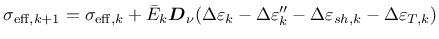(130)

where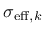is the effective stress vector in the preceding step,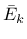is the incremental stiffness,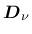is a unit stiffness matrix and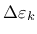,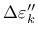,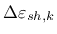,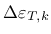are the increments of the total strain, strain due to creep, shrinkage strain (sum of drying and autogenous shrinkage) and thermal strain, respectively.

2. Compute principal effective stresses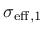,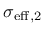,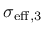3. Evaluate equivalent deformation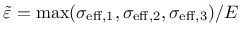(131)

4. If the stress exceeds the material strength, initialize and fix the fracture parameters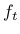and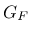(exponential softening assumed).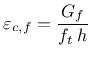(132)

where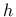is the characteristic length of the finite element in the direction of the biggest principal stress.

5. Evaluate corresponding damage if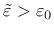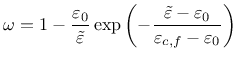(133)

6. Compute principal nominal stresses
• approach #1:
for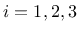if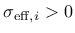,else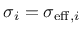• approach #2:
for7. Construct the stress vector in the original configuration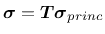(134)

where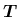is the stress transformation matrix.

The tensile strength and fracture energy can be defined either as fixed, time-independent values (parameters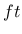,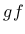) or as variable, depending on the equivalent hydration time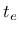. The latter case is activated by keyword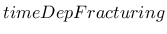; tensile strength and fracture energy are then linked to the current value of the mean compressive strength using (slightly modified) formulae from the fib Model Code 2010.

For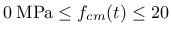MPa the tensile strength is computed from a linear function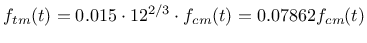(135)

which starts at the origin and at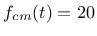MPa connects to expression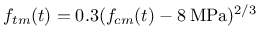(136)

which is valid for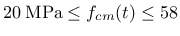MPa. Finally, for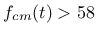MPa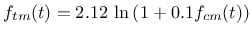(137)

Following the guidelines from the Model Code 2010 (section 5.1.5.2), the fracture energy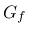can be estimated from the mean compressive strength at 28 days using an empirical formula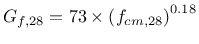(138)

In this function, the mean compressive strength must be provided in MPa and the resulting fracture energy is in N/m.

The growth in fracture energy is approximately proportional to the tensile strength. The current value of fracture energy is very simply computed as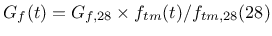(139)

The development of the mean compressive strength in time is adopted entirely from the Model Code 2010 (see section 5.1.9.1)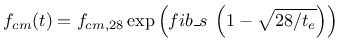(140)

where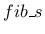is a cement-type dependent coefficient (0.38 for cement 32.5 N; 0.25 for 32.5 R and 42.5 N; 0.2 for 42.5 R and 52.5 N and R),is the equivalent age and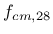is the mean compressive strength at the age of 28 days.

For some concretes the prediction formulae do not provide accurate predictions, therefore it is possible to scale the evolution of tensile strength and fracture energy by providing their 28-day values ft28 and gf28.

The model description and parameters are summarized in Tab. 38.

Table 38: MPS damage-summary.
 Description MPS damage model for concrete creep with cracking (additionaly all parameters from Table 37) Record Format MPSDamMat [ ft(rn) #] [ gf(rn) #] [ timeDepFracturing ] [ fib_s(rn) #] [ ft28(rn) #] [ gf28(rn) #] [ damlaw(in) #] [ isotropic ] [ checksnapback(in) #] Parameters - ft tensile strength (constant in time) - gf fracture energy (constant in time) - timeDepFracturing string activating fib MC 2010 prediction for tensile strength and fracture energy + their time evolution - ft28 manual override for the fib MC 2010 prediction of hydration-dependent tensile strength - gf28 manual override for the fib MC 2010 prediction of hydration-dependent fracture energy - fib_s cement-type dependent coefficient (compulsory with timeDepFracturing) - damageLaw traction-separation law: 0 = exponential softening (default), 1 = linear softening, 6 = damage disabled - isotropic string activating same reduction of stiffness after the onset of cracking both in in compression and tension (enables to use secant stiffness matrix for faster convergence) - checkSnapBack switch for snap-back checking; 1 = activated (default), 0 = deactiavated Supported modes 3dMat, PlaneStress, PlaneStrain

Borek Patzak
2019-03-19